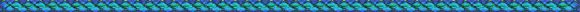Joint Institute for Nuclear Research (JINR)

DUBNA

Е2-2006-17

V. A. Matveev and A. N. Tavkhelidze (INR, RAS, Moscow)
##### THE QUANTUM NUMBER COLOR, COLORED QUARKS AND QCD

(Dedicated to the 40th Anniversary of the Discovery of the Quantum Number Color)

Report presented at the 99th Session of the JINR Scientific Council, Dubna, 19-20 January 2006
###### CONTENTS
Introduction

The quantum number color and colored quarks

Dynamic quark models of hadrons composed of
quasi-free colored quarks

b) The model of quasi-free quarks and the laws
of scaling at high energies

c) Quark counting formulae

Summary

###### INTRODUCTION

At present, the dominant point of view is that all physical phenomena and processes, both terrestrial and cosmological, are governed by three fundamental forces: gravitational, electroweak and chromodynamic. The color charge serves as the source of chromodynamic forces.

In this talk we shall expose the main stages of the early development of the hypothesis of the quantum number color and of colored quarks, put forward under the ideological influence of and in collaboration with N. Bogolubov at the JINR Laboratory of Theoretical Physics. In these works, the concept of color, colored quarks, was introduced for the first time, and a dynamical description of hadrons was given within the framework of the model of quasi-free colored quarks.

Introduction of the quantum number color permitted one to treat colored quarks as real physical objects, constituents of matter. Further, from the color SU(3) symmetry, the Yang-Mills principle of local invariance and quantization of chromodynamic fields gave rise to quantum chromodynamics — the modern theory of strong interactions.

###### THE QUANTUM NUMBER COLOR AND COLORED QUARKS

In 1964, when the hypothesis of quarks — hypothetical particles composing all the observed particles undergoing strong interactions, i.e., mesons and baryons, — was put forward by Gell-Mann and Zweig, quarks were only considered to be mathematical objects, in terms of which it was possible, in a most simple and elegant way, to describe the properties, already revealed by that time, of the approximate unitary SU(3) symmetry of strong interactions. At the beginning, these particles, exhibiting fractional charges and not observable in a free state, were not attributed the necessary physical interpretation.

First of all, making up hadrons of quarks, possessing spin 1/2, led to a contradiction with the Pauli principle and the Fermi-Dirac statistics for systems composed of particles of semiinteger spin.

The problem of the quark statistics was not, however, the sole obstacle in the path of theory. No answer existed to the following question: why were only systems consisting of three quarks and quark-antiquark pairs realized in Nature, and why were there no indications of the existence of other multiquark states?

Especially important was the issue of the possible existence of quarks in a free state (the problem of quark confinement).

In 1965, analysis of these problems led N.Bogolubov, B. Struminsky and A. Tavkhelidze, as well as Y. Nambu and M. Han, and Y. Miyamoto to the cardinal idea of quarks exhibiting a new, hitherto unknown, quantum number subsequently termed color.

From the very beginning, relativistically invariant hadron quark models, unlike the Gell-Mann-Zweig hadron quark model, were dealt with in Dubna, first of all, assuming quarks to be real physical objects determining the structure of hadrons.

To make it possible for quarks to be considered fundamental physical particles, the hypothesis was proposed in 1965 by three authors (Bogolubov, Struminsky, Tavkhelidze) that the quarks, introduced by Gell-Mann and Zweig, should possess an additional quantum number, and that quarks of each kind may exist in three (unitary) equivalent states

q ≡ (q1,q2,q3),

differing in values of the new quantum number, subsequently termed color. Since at the time when the new quantum number was introduced, only three kinds of quarks were known (u, d, s), the quark model with an additional quantum number was termed the three-triplet model. Since the new quantum number is termed color, colored quarks may be in three equivalent states, such as, for example, red, blue and green.

With introduction of the new quantum number, color, the question naturally arose of the possible appearance of hadrons possessing color, which, however, have not been observed. From the assumption that colored quarks are physical objects, while the hadron world is degenerate in the new quantum number, or, as one may customarily say, it is colorless, it followed that solutions of the dynamic equations for baryons and mesons in the s-state should be neutral in the color quantum numbers.

The wave function of the observed hadron family in the ground state, described by the totally symmetric 56-component tensor Фabс(х1,x2,х3) in the approximation of spin-unitary symmetry, was assumed to be totally antisymmetric in the color variables of the three constituent quarks,where A = (а, α), В = (b,β), С = (с, γ), a, b, с are unitary quantum numbers, α, β, γ are color quantum numbers and εαβγ is totally antisymmetric tensor. Hence it is evident that the Pauli principle holds valid for colored quarks, and that they satisfy the Fermi-Dirac statistics, so they can be considered real fundamental constituents of matter.

From the requirement that mesons, composed of a colored quark and an antiquark, be neutral, or colorless, with respect to the new quantum number the meson wave function is chosen in the form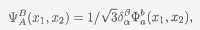where a and b are unitary indices, δβα is Kronecker symbol.

The choice of baryon and meson wave functions, proposed above, leads to the conclusion that the observed mesons and baryons are neutral with respect to the color quantum number, and that the known mesons and baryons are composed of colored quarks and antiquarks as follows: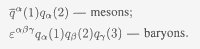Subsequently, the requirement that the world of hadrons be neutral led to the discovery of the SU{3) color symmetry group.

It is to be noted, here, that in his talk delivered to the conference «Symmetry Principles at High Energies», held in Coral Gables (1965), Nambu was the first, on the basis of SU(3) symmetry with respect to the new quantum number (color), to deal with eight vector fields, carriers of the interaction between quarks, which were the prototype of the quantum-chromodynamic gluon fields.

###### DYNAMIC QUARK MODELS OF HADRONS COMPOSED OF QUASI-FREE COLORED QUARKS

The introduction of colored quarks, representing physical fundamental particles, paved the way for the dynamic description of hadrons.

The main obstacle, here, was the absence of quarks in a free state. Although it was evident that the issue of confinement could be ultimately settled only by experiments, a series of attempts was undertaken to provide a logically non-contradictory explanation for the «eternal confinement» of quarks inside hadrons. Thus, for example, P. Bogolubov proposed the «quark-bag» model, known as the Dubna bag. Later, the idea of a quark bag underwent development at MIT, and the resulting model is known as the MIT bag. The dynamic relativistic quark model, the development of which was initiated in Dubna in 1965, was based on the assumption of quarks being extremely heavy objects bound in hadrons by enormous scalar forces, which on the one hand provide for the large quark mass defect in hadrons and on the other hand impede their leaving the hadron.

The dynamic equations were required to have solutions for hadrons, inside which quarks are in a quasi-free state, resulting in the property of approximate additivity, inherent in non-relativistic quark models, being conserved in the calculations of various physical quantities.

In the model of quasi-free quarks the meson wave function represents a second-order mixed spinor ФBA(р) that satisfies the free Dirac equationswhere mq is the effective mass of the quark, and antiquark, in the meson.

The wave function of a baryon composed of three quasi-free quarks represents a third-order mixed spinor ФABC(p) tnat satisfies the equations: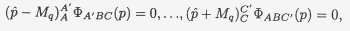where Mq is the renormalized effective mass of the quark in the hadron.

Baryons ФABC and mesons ФBA are represented by a superposition of all admissible states over the quantum numbers (A, B, C) and (A, B), satisfying the requirements of SU(6) symmetry, of quark statistics and of hadron neutrality in the color quantum number.

The dynamic composite quasi-free quark model has made possible the systematic description of both the statically observed characteristics of hadrons (µ, gA /gV, etc.) and their form factors. We introduce weak and electromagnetic interactions in a minimal manner,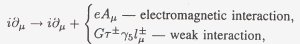where Aµ is the electromagnetic potential, l±µ represent charged lepton weak currents, G is the Fermi weak interaction constant.

For the ratio gA /gV (of the axial and vector weak interaction constants) and for the magnetic moment of the proton we obtain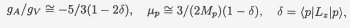where Lz is the orbital momentum of a quark bound in the nucleon with the projection of its total angular momentum equal to 1/2; δ characterizes the magnitude of relativistic corrections and amounts to δ ~ 1/6, the resulting correction for the ratio gA /gV is of the order of 30%. This example shows how significant the effect can turn out to be of relativistic corrections to predictions of the non-relativistic quark model.

The quasi-free quark model has permitted one to explain the lepton decays of pseudoscalar π and К mesons and, also, the electromagnetic decays of the vector mesons into electron-positron pairs as annihilation of quark-antiquark pairs bound in the mesons.

Analysis of the data on the widths of these decays resulted in a conclusion on the dependence of the scales of distances (effective sizes) on the quantum numbers of a bound system, for example,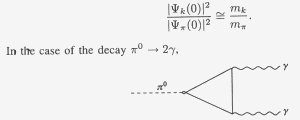determined by the triangular anomaly of the axial current (J. Bell, R. Jackiw, 1969; S. Adler, 1970), the annihilation model points to the width of this decay being proportional to the number of different quark colors (Matveev, Struminsky, Tavkhelidze, 1965).

b) The Model of Quasi-Free Quarks and the Laws of Scaling at High Energies

Experiments, in which inclusive reactions were studied at high energies and momentum transfers, and the scaling regularities revealed, as well as their theoretical investigation, have extended our comprehension of the nature of strong interactions and have given an impetus to further development of the theory of hadron quark structure.

We note, here, that inclusive reactions were first introduced and studied theoretically by Logunov, Nguyen Van Hieu and Mestvirishvili (1967). These results were reported by Logunov at the Rochester conference (1967).

Here, of essential significance was the investigation of deep-inelastic processes in the inclusive scattering of electrons off nucleons, performed at the Stanford center, which in 1968 resulted in observation of the scaling properties of reactions — Bjorken scaling indicating the existence of a «rigid» pointHke nucleonic structure.

Subsequent experimental studies of the scaling properties of inclusive hadron reactions carried out at Protvino (1969), and also of processes of deep-inelastic neutrino and antineutrino interactions with nucleons (CERN, Geneva; FNAL, Batavia) confirmed the idea of the pointlike behavior of the nucleon.

In 1969, on the basis of the quasi-free quark model, the assumption was put forward by Matveev, Muradyan and Tavkhelidze that the scaling properties of electron-nucleon interaction processes, revealed in experiments, are common for all deep-inelastic lepton-hadron processes and that they can be derived in a model-independent manner on the basis of the automodelling principle, or the principle of self-similarity.

The essence of the self-similarity principle consists in the assumption that in the asymptotic limit of high energies and large momentum transfers the form factors and other measurable quantities of deep-inelastic processes are independent of any dimensional parameters (such as particle masses, the strong interaction radius, etc.), which may set the scale of measurement of lengths or momenta. Thus, the form factors of deep-inelastic processes turn out to be homogeneous functions of relativistically invariant kinematic variables, the degree of homogeneity of which is determined by analysis of the dimensionality (it is a key property of conformal invariant theories).

Application of the self-similarity principle for establishing the asymptotic behaviour of the form factors W1(q2,ν) and W2(q2,ν) of deep-inelastic scattering of electrons on protons in the Bjorken regionresults in the Bjorken asymptotic formulae derived in 1968 on the basis of certain assumptionsApplication of the self-similarity principle resulted in the scaling law being found for the first time (Matveev, Muradyan, Tavkhelidze, 1969), which describes the mass spectrum of muon pairs, produced in inclusive proton collisions,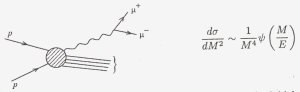where M is the effective mass of the muon pair, and E is the initial energy of the colliding particles. Later, this process was called Drell-Yan process (1970).

Experimental studies of this process, initiated in 1970 by the group of L. Lederman at Brookhaven, confirmed this scaling law, and it was precisely in these processes that a new class of hadrons, theJ/φ particles, was subsequently observed.

c) Quark Counting Formulae

In the case of binary reactions a+b → c+d at high energies s and momentum transfers t, application of the self-similarity principle yields for the differential cross section the following formula of the quark counting (Matveev, Muradyan, Tavkhelidze, 1973)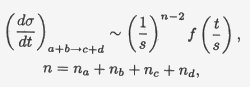where n is the total number of quarks belonging to the particles participating in the reactions. If particle b, for instance, is a lepton, then nb = 1 and one obtains the asymptotic formula for the baryon form factor.

The function ƒ(t/s) depends only on the relation between large kinematic variables and is itself a dimensional quantity. Thus, the asymptotic power law points to factorization of the effects of large and small distances.

###### SUMMARY

We described the scaling properties of elementary particle interaction processes observed experimentally at high energies and large momentum transfers on the basis of the self-similarity principle.

At the same time the question arises concerning the extent to which scaling invariant behaviour is consistent with the main requirements of local quantum field theory.

In the case of deep-inelastic electron scattering on nucleons, these problems were investigated by Bogolubov, Vladimirov and Tavkhelidze (1972) for form factors, which in the Bjorken region have the asymptote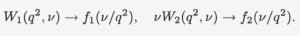For the weight functions of these form factors in the Jost-Lehman-Dyson representation, sufficient conditions were found that guarantee Bjorken scaling. In the case of free quarks the weight functions automatically satisfy these restrictions, which is precisely what provides for Bjorken scaling in the quasi-free quark model.

Note that the subsequent discovery (1973) by Gross, Wilczek and Politzer of the phenomenon of asymptotic freedom in QCD of an invariant charge, introduced by Bogolubov and Shirkov in the renormgroup theory, is an essentially important step for substantiation of the picture of quasi-free quarks in hadrons.

In a number of important works of the last years ('t Hooft, Maldacena, Polchinski, Polyakov, Witten) there was suggested an impressive non-perturbative derivation of the asymptotic power laws (the quark counting for form factors and the exclusive scattering cross sections of hadrons) in the framework of the conformal versions of QCD dual to the string theory.

The power law, which is confirmed in many different experiments and predicted by various model considerations, is the fundamental law of the Nature in the quark physics of hadrons, still needs further deep theoretical investigations.

The references to the original papers mentioned in the talk are presented in the recently published JINR Preprint D2-2005-164 (V. A. Matveev and A. N. Tav-khelidze. The Quantum Number Color, Colored Quarks and QCD (Dedicated to the 40th Anniversary of the Discovery of Color)).

P.S. We looked through D. Gross's Nobel Prize lecture (Uspekhi Fizicheskikh Nauk, 175 (2005) 1306) with interest. Our recent JINR preprint «The Quantum Number Color, Colored Quarks and QCD (Dedicated to the 40th Anniversary of the Discovery of Color)» (D2-2005-164) and the latest talk given at the 99th Session of the JINR Scientific Council can supplement the picture of this lecture with due regard for the priority research achievements made in the opposite hemisphere of the globe.